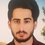# Divergence of Infinite Series of Periodic Functions

We all know that sums of periodic function are divergent. Take a look at this series:

$\displaystyle S=\sum_{n=0}^{\infty} \cos n$

It is divergent yes, but look at the following manipulations:

$\displaystyle = \Re \sum_{n=0}^{\infty} e^{in}$

$\displaystyle = \Re \frac{1}{1-e^i}$

$\displaystyle = \frac{\frac{1}{1-e^i} +\frac{1}{1-e^{-i}} }{2}$

$\displaystyle = \frac{1}{2}$

Shouldn't there be a mathematical error in some of the steps?? Analytically, the series should diverge since the summand is periodic and bounded, but what about the calculations??Note by Hasan Kassim
5 years, 10 months ago

This discussion board is a place to discuss our Daily Challenges and the math and science related to those challenges. Explanations are more than just a solution — they should explain the steps and thinking strategies that you used to obtain the solution. Comments should further the discussion of math and science.

When posting on Brilliant:

• Use the emojis to react to an explanation, whether you're congratulating a job well done , or just really confused .
• Ask specific questions about the challenge or the steps in somebody's explanation. Well-posed questions can add a lot to the discussion, but posting "I don't understand!" doesn't help anyone.
• Try to contribute something new to the discussion, whether it is an extension, generalization or other idea related to the challenge.
• Stay on topic — we're all here to learn more about math and science, not to hear about your favorite get-rich-quick scheme or current world events.

MarkdownAppears as
*italics* or _italics_ italics
**bold** or __bold__ bold
- bulleted- list
• bulleted
• list
1. numbered2. list
1. numbered
2. list
Note: you must add a full line of space before and after lists for them to show up correctly
paragraph 1paragraph 2

paragraph 1

paragraph 2

[example link](https://brilliant.org)example link
> This is a quote
This is a quote
    # I indented these lines
# 4 spaces, and now they show
# up as a code block.

print "hello world"
# I indented these lines
# 4 spaces, and now they show
# up as a code block.

print "hello world"
MathAppears as
Remember to wrap math in $$ ... $$ or $ ... $ to ensure proper formatting.
2 \times 3 $2 \times 3$
2^{34} $2^{34}$
a_{i-1} $a_{i-1}$
\frac{2}{3} $\frac{2}{3}$
\sqrt{2} $\sqrt{2}$
\sum_{i=1}^3 $\sum_{i=1}^3$
\sin \theta $\sin \theta$
\boxed{123} $\boxed{123}$

## Comments

Sort by:

Top Newest

This is because the geometric series will converge for $|x| <1$. The fact that you have assumed convergence and applied the formula is causing the anomaly.

- 5 years, 10 months ago

Log in to reply

Now look at this:

$\displaystyle \sum_{n=1}^{\infty} \frac{\cos n}{n}$

$\displaystyle = \Re \sum_{n=1}^{\infty} \frac{e^{in}}{n}$

$\displaystyle = -\Re \ln (1-e^{i})$

$\displaystyle = -\frac{\ln (1-e^{i}) +\ln (1-e^{-i}) }{2}$

$\displaystyle = -\frac{1}{2} \ln (2-2\cos 1)$

Now this series Converges to this value(you can check by wolfram alpha) .we know that the sum $\sum_{n=1}^{\infty} \frac{x^n}{n}$ converges iff $|x|<1$ . But I used the same $x = e^i$ used in the periodic sum. How can that be justified??

I mean if we say that $|e^i| = 1$, this will contradict the convergence of $\sum_{n=1}^{\infty} \frac{\cos n}{n}$.

- 5 years, 10 months ago

Log in to reply

It is not true that $\sum \frac { x^n } { n }$ converges iff $|x| < 1$.
The proper version of the statement is that $\sum \frac { x^n } { n }$ converges absolutely iff $|x| < 1$.

For example, we know that $\sum \frac{ (-1)^n} { n}$ converges conditionally to $\ln 2$.

(I believe that) we get conditional convergence if we substitute $|x| = 1, x \neq 1$. In particular, $x = e^i$ is valid.

Staff - 5 years, 10 months ago

Log in to reply

Okay I got it.Thanks for the insight :)

Do you know a regularization to this series? "Assigning values to divergent series"?

And in General, for what reasons we assign values to divergent series? and doesn't that make any contradiction?

- 5 years, 10 months ago

Log in to reply

Any Thoughts??

- 5 years, 10 months ago

Log in to reply

×

Problem Loading...

Note Loading...

Set Loading...